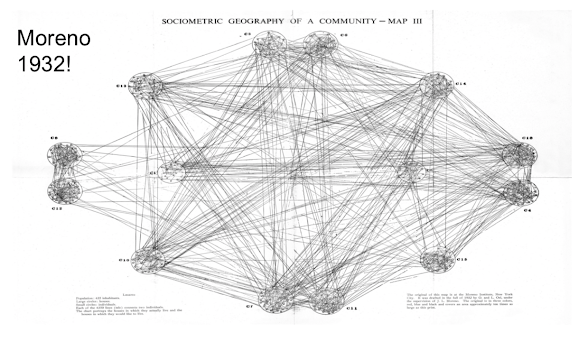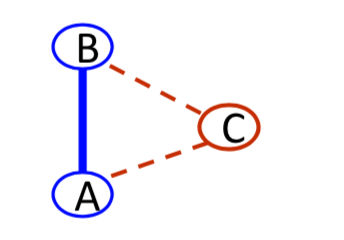# 3.7 ERGMs¶

Models of networks that allow us to capture interdependencies, these are often known as exponential random graph model

## Random Network Models:¶

• Erdos-Renyi
• Useful for understanding thresholds and how networks come to exhibit certain features
• Miss many real-world features: e.g., clustering
• Watts and Strogatz, Barabasi and Albert, Jackson and Rogers...
• Capture other features: clustering, degree distribution, correlation...
• Stochastic Block Models
• Enrich Erdos-Renyi to allow for probabilities to depend on node characteristics, attributes(or on latent - unobserved characteristics)
• Popular set of models: ERGMs and new ones: SERGMs/SUGMs
• flexible way to introduce various local features and dependencies
• estimated statistically

A pertinent form of statistical treatment would be one which deals with social configurations as wholes, and not with single series of facts, more or less artificially separated from the total picture.

Jacob Levy Moreno and Helen Hall Jennings, 1938

We're looking at social interactions and people are interrelated, we're not going to be able to look at just binary relationship diads.## Markov, $P^*$, ERGMs¶

• The previous models not sufficient for fitting data with clustering or other dependencies or testing many social / economic theories
• Link ij's probability could depend on presence of jk and ik
• The link between two individuals i and j could depend on the presence of a friend in common. Does j know k and i know k as well? That's something we want to capture, the difficult with this is, once we allow one link to depend on another link, then we open a Pandora's box, where now all the links could be inter-dependent. So everything gets put back together and so we have to specify all of the interdependencies.
• But then things interlock and need to specify full interdependencies
• Frank and Strauss(1986) $P*$ models (e.g. Wasserman and Pattison(1996))

# p* and ERG Models¶

• Example: (studied extensively by Strauss (86), Park and Newman (04,05), Chatterjee, Diaconis (11)...)

• Probability of a network depends on number of links

• Probability of a network also depends on number of triangles.

# Example¶

• Likelihood of link depends on node attributes

• also depends on whether nodes have friends in common## ERGMs¶

Example: probability depends on: $\beta_LN_{links(g)} + \beta_TN_{triangles(g)}$

Want probability of network to depend on $\beta_LL(g) + \beta_TT(g)$

Exponentiate this formula($\beta_LL(g) + \beta_TT(g)$), so it's always going to be non-negative, it's a standard trick in statistics work with the exponential family:

Set $Pr(g)$ ~ $exp^{\beta_LL(g) + \beta_TT(g)}$

Theorem by Hammersly and Clifford(71): any network model can be expressed in the exponential family with counts of graph statistics.

## Example: Erdos-Renyi G(n, p)¶

• p is the probability of a link, L(g) is the number of links in a given graph g

$Pr[(g)] = P^{L(g)}(1-P)^{n(n-1)/2 - L(g)}$

$= (p/(1-p))^{L(g)}$

$= exp(log(p / (1-p))L(g) - log(1 / (1 - p))n(n-1) / 2)$

$= exp( \beta_1 S_1(g) - c)$

What it does give us an idea that you can convert a lot of other kinds of models into the exponential random graph family.

If one fits an ERGM G(n,p) with just links, and finds a parameter $\beta_1 = 0.5$.

Based on $\beta_1 = log(p/(1 - p))$, hence:

$p = e^{\beta_1} / (1 + e^{\beta_1})$

$= e^5 / (1 + e^5)$

## ERGMs¶

To be probability:

$Pr(g) = \frac{exp(\beta_LL(g) + \beta_TT(g))}{\sum_{g'}exp(\beta_LL(g') + \beta_TT(g'))}$

Now one other thing to make this probability of these have to sum to 1, so in particular that means that We have to normalize by what the probability of all the graphs are($\sum_{g'}exp(\beta_LL(g') + \beta_TT(g'))$), to make sure the probability of one particular graph when we sum across all the graphs, this is going to sum up to one.

$Pr(g) = exp(\beta_LL(g) + \beta_TT(g) - c)$